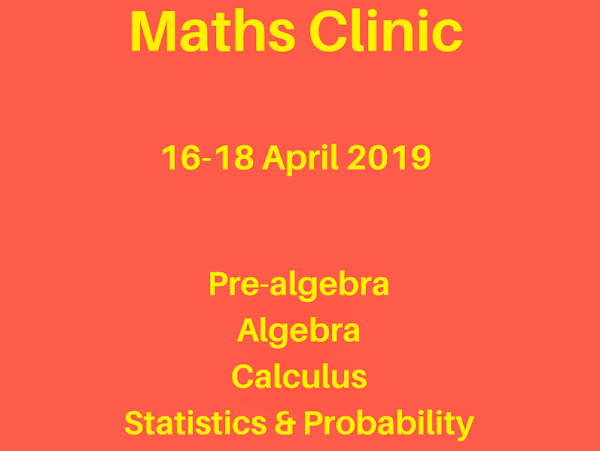Posted on Mar 20, 2019

# Teacher Penelope

## Maths Clinic

Apr 16, 2019 – Apr 18, 2019
For those keen on advancing their maths skills, I am opening a Maths clinic on 16-18 April: 2-hr sessions that involve working on as many maths questions as possible while discussing maths concepts. Book by 31 March.

There are 4 streams. Book 1 or more sessions to brush up on your maths.

1. Pre-algebra
2. Algebra
3. Calculus
4. Statistics & probability

TIMETABLE

16 April, Tuesday

9:30am-11:30am Pre-algebra 1: Numbers & Arithmetic
-sets, operations on sets, the real number system, properties of operations, integers, fractions, decimals, percentages

-roots & powers, laws of exponents, prime & composite, factorisation, bidmas, areas, volumes

2:30pm-4:30pm Algebra 1: Beginning algebra
-algebraic expressions, operations & simplifying expressions, laws of exponents, factoring

17 April, Wednesday

9am-11am Algebra 2: Intermediate algebra
-equations, relations & functions, linear equations, simultaneous equations

11.30am-1:30 Calculus 1: Beginning calculus
- limit of a function, derivatives, graphing functions

2pm-4pm Statistics and probability: Beginning statistics
- descriptive statistics, visualising data & calculating simple probability

18 April, Thursday

10:30am-12.30pm Calculus 2: Intermediate Calculus
- more on derivatives, antiderivative/integrals